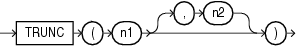## TRUNC (number)

Syntax

trunc_number::=Description of the illustration trunc_number.eps

Purpose

The `TRUNC` (number) function returns `n1` truncated to `n2` decimal places. If `n2` is omitted, then `n1` is truncated to 0 places. `n2` can be negative to truncate (make zero) `n2` digits left of the decimal point.

This function takes as an argument any numeric data type or any nonnumeric data type that can be implicitly converted to a numeric data type. If you omit `n2`, then the function returns the same data type as the numeric data type of the argument. If you include `n2`, then the function returns `NUMBER`.

Examples

The following examples truncate numbers:

```SELECT TRUNC(15.79,1) "Truncate" FROM DUAL;

Truncate
----------
15.7

SELECT TRUNC(15.79,-1) "Truncate" FROM DUAL;

Truncate
----------
10```